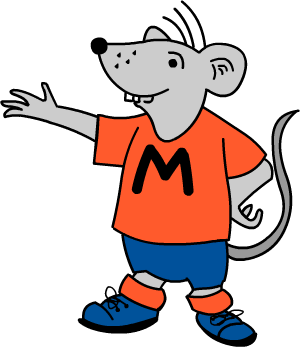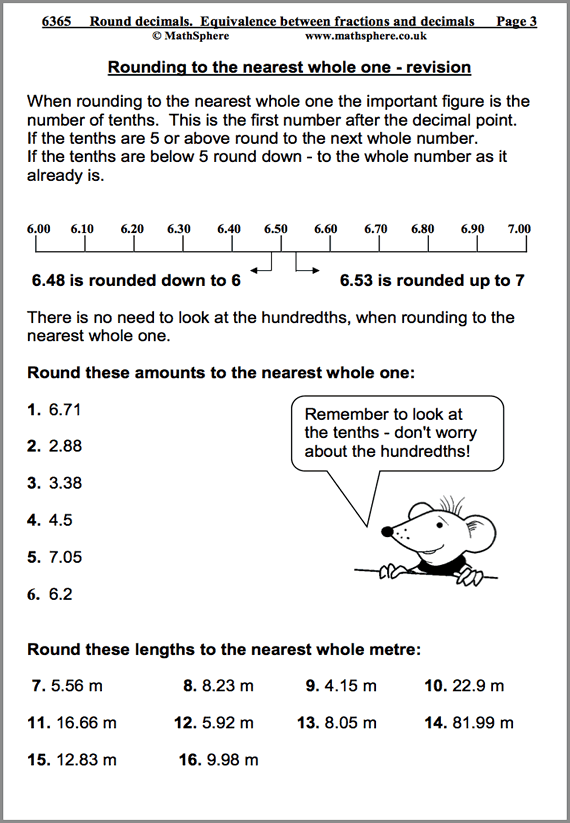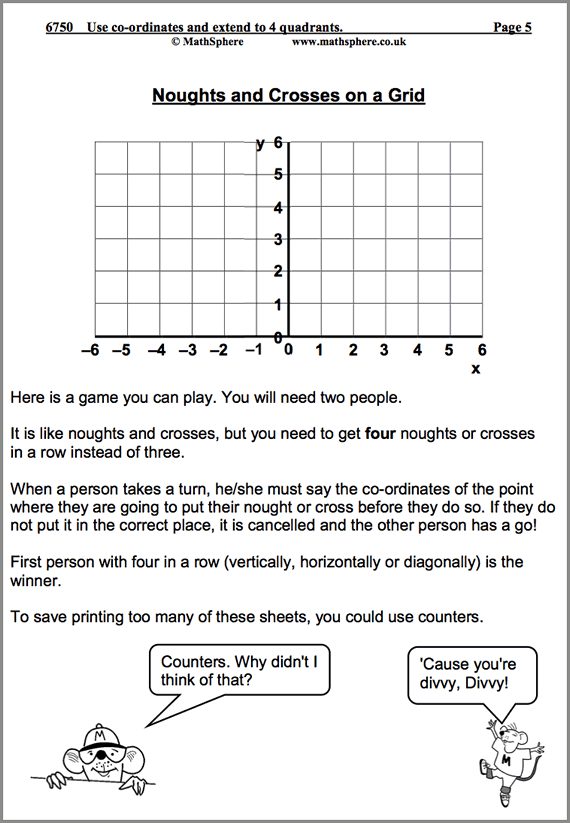# Year 6 Maths Worksheets

A great range of worksheets for the oldest primary children in Year 6 (aged 10‐11). MathSphere is the trusted home of quality maths worksheets, whether to use in the classroom, as back-up material or for homework.

## The Very Best Year 6 Maths Worksheets

The new curriculum is here and we have updated our resources to ensure that the challenges ahead can be met confidently and successfully. Our worksheets help children become proficient in all areas of Maths including algebra and statistics.

• Updated for the very latest statutory requirements with many new pages added
• Answers included to make marking simple
• Concept pages clearly explain the ideas being taught
• Over 800 high quality pages of questions and answers
• Single user licence for teachers or parents (school site licence upgrade available)
• MathSphere has been a favourite resource for Year 6 teachers for over 15 years## Try Some Free Sample Worksheets

To give you a better idea of the worksheets available we have provided a selection of free modules for Year 6.

Please download these samples, print them out and use at home, or photocopy and use in the classroom.28 Pages

#### Rounding Decimals

Understanding the relationship between decimals and fractions is crucial for future success.10 Pages

#### Conventions For Working Out Expressions

Calculating in the correct order: BODMAS as it is often known.13 Pages

#### Use Co-Ordinates & Extend into 4 Quadrants

Great fun, but important to understand for future work at High School.

## List of modules

It is impossible to describe in a single page the massive amount of high quality maths contained in this one product.

To give you some idea, have a look at the list of all modules:

### Number and Place Value 43 pages

 Read and write very large numbers. 5 Revise rounding whole numbers. 12 Recognise and order negative numbers. 17 Revise estimating and approximating. 9

### Fractions 149 pages

 Use fraction notation.Recognise equivalent fractions. 22 Find fractions of numbers or quantities. 9 Adding fractions. 8 Fraction investigation 1. 3 Investigate halves. 3 Subtracting fractions. 12 Multiplying and dividing fractions. 7 Use decimal notation. Order decimal fractions. 14 Use known facts to multiply and divide decimals. 14 Round decimals. Equivalence between decimals and fractions. 28 Understand remainders. Round up or down. 17 Percentages. 12

### Algebra 52 pages

 Make and investigate general statements. 13 Extend number sequences. 12 Algebra. 24 Algebra investigation. 3

### Geometry 86 pages

 Visualise, describe and classify 3D and 2D shapes. 8 More shapes and nets. 11 More measuring angle. 16 Investigate exterior and interior angles. 6 More properties of shapes. 16 Reflective symmetry. Reflections and translations. 16 Use co-ordinates and extend to four quadrants. 13

### Using and Applying Mathematics 105 pages

 Solve problems involving ‘real life’. 8 Solve problems involving money. 8 Solve problems involving time. 8 More time problems. 8 Choose appropriate methods of calculating. 11 Explain methods and reasoning. 7 Solve problems, generalise and predict. 11 Checking results of calculations. 11 Develop calculator skills. 9 Calculator fractions. 3 Maths vocabulary games. 8 Investigate factors and primes. 7 Investigate four fours. 3 Primes from squares. 3

### 209 pagesAddition, Subtraction, Multiplication and Division

 Revise addition. 16 Revise subtraction. 12 Use known number facts to add and subtract decimals. 11 Relationship between addition and subtraction. Add several numbers. 14 Revise multiplication. 13 Use known facts to multiply mentally. 19 Revise division. 12 Revise doubling and halving. Using factors. 14 Square numbers, prime numbers and identifying factors. 15 Use formal written methods of addition. 10 Use formal written methods of subtraction. 16 Use formal written methods of multiplication. 6 Long multiplication. 8 Use formal written methods of long division. 5 More long division: 3-digits divided by 2-digit numbers. 8 Division with decimals. 8 Multiples, factors and tests of divisibility. 12 Conventions for working out expressions. (Bodmas) 10

### Ratio and Proportion 53 pages

 Begin to understand ratio and proportion. 11 Solve problems concerning ratio and proportion. 9 Ratio and proportion problems. 12 Scale/Proportion/Conversion of units. 13 Scale drawing. 8

### Measurement 93 pages

 Use standard metric units and convert. 8 Units to estimate and measure length, mass and capacity. 8 Solve measurement problems. 7 Read scales, record estimates and measure. 14 Measure and calculate the perimeter and area of simple shapes. 15 More perimeter and area. 25 Measure and calculate the circumference of circles. 11 Farmer's field investigation. 5

### Statistics 37 pages

 Collect, sort and organise data. Pie charts. 11 Further data handling. 11 Probability. 9 Investigate measurement statistics. 6

### Mental arithmetic 75 pages

 Autumn term. 25 Spring term. 25 Summer term. 25

### Quick Details

• Over 800 worksheets